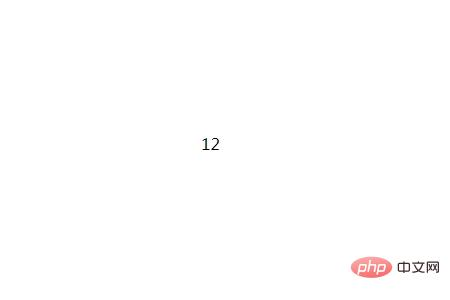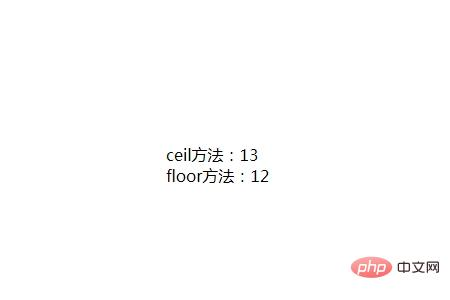# JavaScript如何实现数据的四舍五入round()方法：可把一个数字舍入为最接近的整数，即四舍五入

toFixed()方法：可把 Number 四舍五入为指定小数位数的数字。

parseInt()方法：可将小数转化为整数（位操作符）

ceil()方法：可对一个数进行上舍入

floor()方法：可对一个数进行下舍入

round()方法

```	document.write(Math.round(4.55) + "<br>");
document.write(Math.round(-6.45));```toFixed()方法

```var num=3.141592;
document.write(num.toFixed(2));```parseInt()方法

```document.write(parseInt("12.333"));
```ceil()方法与floor()方法

```document.write("ceil方法：")
document.write(Math.ceil("12.333") + "<br>");
document.write("floor方法：")
document.write(Math.floor("12.333"));```本文地址：http://itbyc.com/web/javascript/20148.html